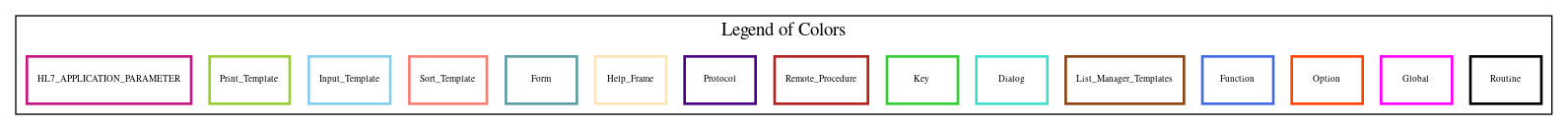Home   Package List   Routine Alphabetical List   Global Alphabetical List   FileMan Files List   FileMan Sub-Files List   Package Component Lists   Package-Namespace Mapping
Routine: OOPSGUIR

# Routine: OOPSGUIR

## Information

OOPSGUIR ;WIOFO/LLH-RPC routine for misc reports ; 6/11/09 10:32am

## Source Information

Source file <OOPSGUIR.m>

## Call Graph### Call Graph Total: 7

Package Total Call Graph
Asists 4 STATINFO^OOPSGUI3  SITEPGET^OOPSGUI6  DSPUTE^OOPSGUIF  (CMPLSRV,DSPUTE,SERVICE)^OOPSGUIU
VA FileMan 2 ^%DT  \$\$GET1^DIQ
Kernel 1 (\$\$FMDIFF,\$\$FMTE,\$\$HTFM)^XLFDT

## Caller Graph

### Legends:#### Package Component Superscript legend

 action A extended action Ea event driver Ed subscriber Su protocol O limited protocol LP run routine RR broker B edit E server Se print P screenman SM inquire I### Caller Graph Total: 1

Package Total Caller Graph
Asists 1 OOPS GET MISC REPORT DATA

## Entry Points

ENT(RESULTS,INPUT,CALL) ; get the data for the report
; Input: INPUT - contains 3 values, the START AND END DATE,
; STATION. The Date of Occ (fld #4) is used to
; in/exclude claims from the report. If Station='ALL'
; then all claims are included, if not 'All', then
; only 1 station is included.
; CALL - contains the report call which will invoke
; the appropriate M call
; Output: RESULTS - the results array passes data back to the client.
SERVICE ; Service/Detail Location report - patch 11
DSPUTE ; Reason for Dispute report. Patch 11
FLD174 ; Report compiles filing instruction result counts
FLD332 ; Use this tag for Reason for Controvert report. Patch 11
SUM300A ; Summary of Work-related injuries and illness report
IRWSHT ; Incidence Rates Worksheet Report
DETAIL ; now get employee information
LOG300 ; entry point for the OSHA 300 LOG
FLD95 ; use OUTC subrecord to retrieve data
EMPHRS ; get Total Num Employees and Hours worked

## External References

Name Field # of Occurrence
^%DT ENT+17, ENT+18
\$\$GET1^DIQ FLD332+13, FLD332+14, FLD332+23, FLD332+24, FLD332+29, FLD332+32, FLD332+33, FLD332+37, SUM300A+6, LOG300+12
LOG300+13, LOG300+14, LOG300+17, LOG300+18, LOG300+19, LOG300+20, LOG300+22, LOG300+24
STATINFO^OOPSGUI3 SUM300A+7
SITEPGET^OOPSGUI6 SUM300A+13
DSPUTE^OOPSGUIF FLD332+19
CMPLSRV^OOPSGUIU FLD332+47
DSPUTE^OOPSGUIU FLD332+48
SERVICE^OOPSGUIU FLD332+20
\$\$FMDIFF^XLFDT FLD95+16, FLD95+17
\$\$FMTE^XLFDT LOG300+19
\$\$HTFM^XLFDT FLD95+4

## Used in RPC

RPC Name Call Tags
OOPS GET MISC REPORT DATA ENT

## FileMan Files Accessed Via FileMan Db Call

FileNo Call Tags
^OOPS(2260 - [#2260] GET1^DIQ
^DIC(4 - [#4] GET1^DIQ

## Global Variables Directly Accessed

Name Line Occurrences  (* Changed,  ! Killed)
^DD(2260 FLD332+4
^DIC(5 - [#5] SUM300A+10, SUM300A+11
^OOPS(2260 - [#2260] FLD332+11, FLD332+12, FLD332+16, FLD332+35, LOG300+3, LOG300+4, LOG300+5, LOG300+6, LOG300+9, FLD95+2
FLD95+3, FLD95+7, FLD95+8
^OOPS(2262 - [#2262] EMPHRS+5, EMPHRS+16, EMPHRS+17
^OOPS(2262.4 - [#2262.4] FLD332+8, FLD332+43
^TMP(\$J ENT+15!, FLD332+44*, FLD332+46*, FLD332+49, SUM300A+5*, SUM300A+12*, SUM300A+19*, IRWSHT+2*, LOG300+27*, LOG300+28*
LOG300+32*, LOG300+33

## Label References

Name Line Occurrences
@( ENT+22
DETAIL SUM300A+21, IRWSHT+5
EMPHRS SUM300A+21, IRWSHT+5
FLD95 LOG300+10, LOG300+11

## Local Variables

### Legend:

 >> Not killed explicitly * Changed ! Killed ~ Newed

Name Field # of Occurrence
%DT ENT+9~
ARR FLD332+1~, SUM300A+7!, SUM300A+13!, SUM300A+20!, IRWSHT+4!, LOG300+34!
ARR( FLD332+5*, FLD332+6*, FLD332+8*, FLD332+25*, FLD332+34*, FLD332+41, FLD332+44, FLD332+46, SUM300A+14, SUM300A+15
SUM300A+16, SUM300A+17, SUM300A+18
ARR("999:Case not controverted,  no controvert code expected" , FLD332+30*, FLD332+31*
ARR(0 SUM300A+8, SUM300A+11*, SUM300A+12
ARR(1 LOG300+12*, LOG300+25
ARR(10 LOG300+26, FLD95+39*, FLD95+40*, FLD95+41*
ARR(2 LOG300+12*, LOG300+13*, LOG300+15*, LOG300+16*, LOG300+25
ARR(3 LOG300+18*, LOG300+25
ARR(4 LOG300+19*, LOG300+25
ARR(5 LOG300+20*, LOG300+25
ARR(6 LOG300+22*, LOG300+23*, LOG300+24*, LOG300+25
ARR(7 LOG300+25, FLD95+39*, FLD95+43*, FLD95+44*, FLD95+45*, FLD95+46*
ARR(8 LOG300+26, FLD95+39*, FLD95+42*, FLD95+43*
ARR(9 LOG300+26, FLD95+39*, FLD95+42*, FLD95+43*
ARR(98 FLD332+9*, FLD332+38*
ARR(99 FLD332+10*
AVAIL FLD95+1~, FLD95+20*, FLD95+21*, FLD95+24*, FLD95+25*, FLD95+26, FLD95+27
CALL ENT~, ENT+12
CASES LOG300+1~, LOG300+2*, LOG300+7*, LOG300+28, LOG300+30, EMPHRS+1~
CAX ENT+9~, FLD332+14*, FLD332+15
CN FLD332+1~, FLD332+2*, FLD332+40*, FLD332+42*, FLD332+44, FLD332+46, SUM300A+1~, SUM300A+14*, SUM300A+15, SUM300A+16
SUM300A+17, SUM300A+18, LOG300+1~, LOG300+2*, LOG300+27*
CODE FLD332+1~, FLD332+4*, FLD332+5, FLD332+42*, FLD332+43*, FLD332+44
COLF LOG300+1~, LOG300+22*, LOG300+23, LOG300+24
COLG SUM300A+2~, SUM300A+3*, LOG300+30, FLD95+34*
COLH SUM300A+2~, SUM300A+3*, LOG300+30, FLD95+35*
COLHI IRWSHT+1~, IRWSHT+3*, LOG300+9*, LOG300+28
COLI SUM300A+2~, SUM300A+3*, LOG300+30, FLD95+36*
COLJ SUM300A+2~, SUM300A+3*, LOG300+30, FLD95+37*
COLK SUM300A+2~, SUM300A+3*, LOG300+30, FLD95+33*
COLL SUM300A+2~, SUM300A+3*, LOG300+30, FLD95+33*
COLM SUM300A+2~
COLM( FLD95+31*
COLM(1 SUM300A+4*, LOG300+31, FLD95+29*
COLM(2 SUM300A+4*, LOG300+31
COLM(3 SUM300A+4*, LOG300+31
COLM(4 SUM300A+4*, LOG300+31
COLM(5 SUM300A+4*, LOG300+31
COLM(6 SUM300A+4*, LOG300+31, FLD95+32*
DATA SUM300A+20!*, IRWSHT+4!*, LOG300+25*, LOG300+26*, LOG300+27, LOG300+30*, LOG300+31*, LOG300+32, LOG300+34!
DAYA FLD95+1~, FLD95+6*, FLD95+19, FLD95+21, FLD95+24, FLD95+25, FLD95+26*, FLD95+33, FLD95+42
DAYJ FLD95+1~, FLD95+6*, FLD95+19, FLD95+21, FLD95+24, FLD95+25, FLD95+27*, FLD95+33, FLD95+42
DAYS FLD95+1~, FLD95+9*, FLD95+16*, FLD95+17*, FLD95+18*, FLD95+21, FLD95+22, FLD95+24, FLD95+25
DOI LOG300+1~, LOG300+2*, LOG300+3*, LOG300+4
ED FLD95+1~, FLD95+9*, FLD95+16, FLD95+17, EMPHRS+1~, EMPHRS+9*, EMPHRS+10, EMPHRS+13, EMPHRS+14, EMPHRS+18
EDATE ENT+9~, ENT+16*, ENT+18*, ENT+21*, FLD332+11, LOG300+3, LOG300+23, LOG300+24, FLD95+13, FLD95+16
EMPHRS+9
EMP SUM300A+1~, IRWSHT+1~, LOG300+30, EMPHRS+2*, EMPHRS+20*, EMPHRS+21*, EMPHRS+22*
ENDDT ENT+9~, ENT+11*, ENT+13, ENT+18
FAC SUM300A+1~, SUM300A+6*, SUM300A+12
FI ENT+9~, FLD332+23*, FLD332+24*, FLD332+25, FLD332+32*, FLD332+33*, FLD332+34, FLD332+40*, FLD332+41*, FLD332+42
FLD332+44, FLD332+46
FLD LOG300+1~, LOG300+17*, LOG300+18
GOON FLD332+1~, FLD332+29*, FLD332+37*
HRS SUM300A+1~, IRWSHT+1~, LOG300+28, LOG300+30, EMPHRS+2*, EMPHRS+20*, EMPHRS+22*
I FLD332+1~, FLD332+5*, FLD332+6, FLD332+8*, FLD332+24
IEN FLD332+1~, FLD332+2*, FLD332+12*, FLD332+13, FLD332+14, FLD332+16, FLD332+23, FLD332+24, FLD332+29, FLD332+32
FLD332+33, FLD332+35, FLD332+37, LOG300+1~, LOG300+3*, LOG300+4*, LOG300+5, LOG300+6, LOG300+9, LOG300+12
LOG300+13, LOG300+14, LOG300+17, LOG300+18, LOG300+19, LOG300+20, LOG300+22, LOG300+24, FLD95+2, FLD95+3
FLD95+7, FLD95+8
IEN95 FLD95+1~, FLD95+6*, FLD95+7*, FLD95+8
ILL FLD95+1~, FLD95+3*, FLD95+31, FLD95+32, FLD95+41
INC LOG300+1~, LOG300+17*, FLD95+1~, FLD95+2*, FLD95+29, FLD95+30, FLD95+40, FLD95+41
INPUT ENT~, ENT+11, ENT+12
LP ENT+9~, FLD332+1~, FLD332+2*, FLD332+5*, FLD332+11*, FLD332+12
LV1 EMPHRS+1~, EMPHRS+4*, EMPHRS+5, EMPHRS+16, EMPHRS+17
LV2 EMPHRS+1~, EMPHRS+4*, EMPHRS+5, EMPHRS+16, EMPHRS+17
MON EMPHRS+1~, EMPHRS+15*, EMPHRS+21
OC FLD95+1~, FLD95+9*, FLD95+14, FLD95+18, FLD95+26, FLD95+27
OK EMPHRS+1~, EMPHRS+12*, EMPHRS+13*, EMPHRS+14*, EMPHRS+15, EMPHRS+20*, EMPHRS+21, EMPHRS+22
OUTC FLD95+1~
OUTC( FLD95+14*
OUTC("A" FLD95+35, FLD95+44
OUTC("D" FLD95+34, FLD95+43
OUTC("J" FLD95+36, FLD95+45
OUTC("O" FLD95+37, FLD95+46
P2 FLD332+1~, FLD332+40*, FLD332+42*, FLD332+43, FLD332+44, FLD332+46
PAR EMPHRS+1~, EMPHRS+3*, EMPHRS+4
RESULTS ENT~, FLD332+49*, LOG300+33*
RESULTS(0 ENT+10*, ENT+14*
S0 FLD95+1~, FLD95+2*
S95 FLD95+1~, FLD95+8*, FLD95+9, FLD95+10, FLD95+18
SD FLD95+1~, FLD95+9*, FLD95+13, FLD95+16, FLD95+17, EMPHRS+1~, EMPHRS+8*, EMPHRS+10, EMPHRS+13, EMPHRS+14
EMPHRS+18
SDATE ENT+9~, ENT+16*, ENT+17*, ENT+21*, FLD332+11, LOG300+2, LOG300+23, EMPHRS+7*, EMPHRS+8
SIEN EMPHRS+1~, EMPHRS+5*, EMPHRS+16, EMPHRS+17
STA ENT+9~, ENT+12*, ENT+13, FLD332+17, SUM300A+6, SUM300A+7, SUM300A+15, LOG300+5, EMPHRS+5
STATE SUM300A+1~, SUM300A+8*, SUM300A+9, SUM300A+10*, SUM300A+11
STATION ENT+9~, FLD332+16*, FLD332+17, LOG300+1~, LOG300+5*
STDT ENT+9~, ENT+11*, ENT+13, ENT+17
STR SUM300A+1~, SUM300A+16*, SUM300A+17*, SUM300A+18*, SUM300A+19, EMPHRS+1~, EMPHRS+17*, EMPHRS+18, EMPHRS+19, EMPHRS+20
TAG ENT+9~, ENT+12*, ENT+13, ENT+15, ENT+20*, ENT+22, FLD332+3, FLD332+7, FLD332+15, FLD332+19
FLD332+20, FLD332+22, FLD332+27, FLD332+39, FLD332+43, FLD332+44, FLD332+46, FLD332+47, FLD332+48, FLD332+49
SUM300A+5, SUM300A+12, SUM300A+19, IRWSHT+2, LOG300+8, LOG300+10, LOG300+11, LOG300+27, LOG300+28, LOG300+29
LOG300+32, LOG300+33, FLD95+13, FLD95+15, FLD95+17, FLD95+28, FLD95+38
>> TAWAY FLD95+6*
TDAY FLD95+1~, FLD95+4*, FLD95+17
TX FLD332+1~, FLD332+43*
TYPE LOG300+1~, LOG300+14*, LOG300+15
U ENT+11, ENT+12, FLD332+4, FLD332+8, FLD332+16, FLD332+43, FLD332+44, FLD332+46, SUM300A+8, SUM300A+11
SUM300A+12, SUM300A+15, SUM300A+16, SUM300A+17, SUM300A+18, SUM300A+19, LOG300+5, LOG300+6, LOG300+25, LOG300+26
LOG300+28, LOG300+30, LOG300+31, FLD95+2, FLD95+3, FLD95+9, FLD95+10, FLD95+18, EMPHRS+18, EMPHRS+19
EMPHRS+20
WS EMPHRS+1~, EMPHRS+2*, EMPHRS+16*, EMPHRS+17
X ENT+9~, ENT+17*, ENT+18*, EMPHRS+1~, EMPHRS+12*
X1 EMPHRS+1~, EMPHRS+10*, EMPHRS+11, EMPHRS+12, EMPHRS+14
X2 EMPHRS+1~, EMPHRS+10*, EMPHRS+11, EMPHRS+12, EMPHRS+14
Y ENT+9~, ENT+17, ENT+18